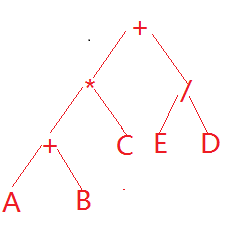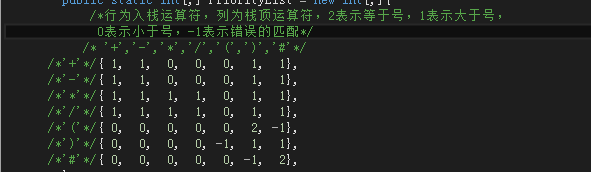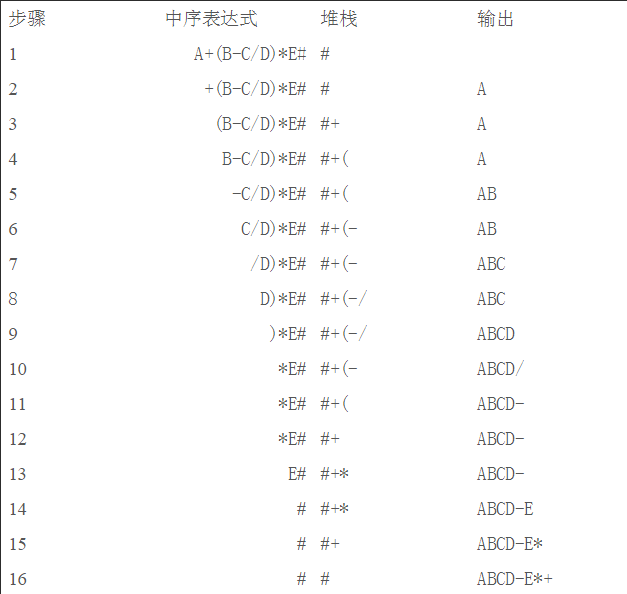• 四则运算算法1.0版（附带小数点识别、正负号识别、运算式错误提示等功能），小数点识别是指当一个数字以小数点开头时，默认它的整数部分为0
• 四则运算算法1.1版（附带小数点识别、正负号识别、运算式错误提示、特殊乘法等功能）。小数点识别是指当一个数字以小数点开头时，默认它的整数部分为0。特殊乘法是指(a)(b)的形式视为a*b来计算。
• 在课余时间写的一个四则运算算法，有很多BUG，练习一下哈……
• 用java模拟解决计算器四则运算算法问题
用java模拟解决计算器四则运算算法问题.
/**
* 模拟实现计算器算法功能
*/
public class Calc {

public static void main(String[] args) {
String exp = "-3*-(3+6/6-3)*1";
System.out.println(calcBracket(exp));
}

/**
* 带括弧的四则运算
* @param exp 带括弧的表达式
* @return
*/
public static double calcBracket(String exp){
//从最里面的括号开始运算,exp中最后出现的(最内层的括号
int lastIndexOf = exp.lastIndexOf('(');
//两种结果
if (lastIndexOf != -1) {
//有
//1.计算最内层括号表达式的值,找对应的右括号
int rightIndexOf = exp.indexOf(')', lastIndexOf);
double d = calc(exp.substring(lastIndexOf+1,rightIndexOf));
//2.把运算结果拼接
exp = exp.substring(0, lastIndexOf)+d+exp.substring(rightIndexOf+1);
return calcBracket(exp);
}else {
//没有
//没有括号的表达式
return calc(exp);
}
}

/**
*
* @param exp 不带括号的四则表达式
* @return
*/
public static double calc(String exp){
//1.把表示负数的-号换成@号
exp = fu2At(exp);
//2.数字的分类
List<Double> numbers = splitNumExp(exp);
//3.运算符的分离
List<Character> ops = splitOpfromExp(exp);
//4.先乘除
for (int i = 0; i < ops.size(); i++) {
//判断,运算符是否是乘除
char op = ops.get(i);
//是,取出,运算
if (op == '*' || op == '/') {
//取出来,运算
ops.remove(i);//后面的数据往前顺序移动
//运算
//从数字容器中取出对应运算符的两个数字
double d1 = numbers.remove(i);
double d2 = numbers.remove(i);

if (op == '*') {
d1 *= d2;
}else {
d1 /= d2;
}

//把运算结果放入数字容器中i的位置
i--;
}
}
//5.后加减
while (!ops.isEmpty() ) {
char op = ops.remove(0);
double d1 = numbers.remove(0);
double d2 = numbers.remove(0);
//运算
if (op == '+') {
d1 += d2;
} else {
d1 -= d2;
}
//把运算结果插入到数字容器中0的位置
}
//6.容器中的第一个数据就是结果
return numbers.get(0);
}

/**
* 从表达式中分离表达式和运算符
* @param exp
* @return
*/
private static List<Character> splitOpfromExp(String exp) {
List<Character> ops = new ArrayList<>();
StringTokenizer st = new StringTokenizer(exp, "1234567890.@");
while (st.hasMoreTokens()) {
char c = st.nextElement().toString().trim().charAt(0);
}
return ops;
}

/**
* 分离出数字
* @param exp
* @return
*/
private static List<Double> splitNumExp(String exp) {
List<Double> numbers = new ArrayList<>();
StringTokenizer st = new StringTokenizer(exp, "+-*/");
while (st.hasMoreTokens()) {
String numStr = st.nextElement().toString().trim();
if (numStr.charAt(0) == '@') {
numStr = "-" + numStr.substring(1);
}
}
return numbers;
}

/**
* 把-号换成@号
* @param exp
* @return
*/
private static String fu2At(String exp) {
for (int i = 0; i < exp.length(); i++) {
char c = exp.charAt(i);
if (c == '-') {
//判断是否是负数
if (i == 0) {
//第一个位置肯定是负数
exp = "@"+exp.substring(1);
}else {
//不是第一个位置
//判断前一个位置是否是运算符
char cprev = exp.charAt(i - 1);
if (cprev == '+' || cprev == '-' || cprev == '*' || cprev == '/') {
exp = exp.substring(0, i)+"@"+exp.substring(i+1);
}
}
}
}
return exp;
}
}

展开全文数据结构 计算器
• 算法采用值栈，符号栈，以及左右括号，乘除，加减优先等级设置方式实现运算
• 用C++编写的两个数四则运算算法。 其中海包括除数不能为零的情况，是一种较简单的算法~C++
• 这个程序有点错；请下另一个；不好意思；呵呵 把乘法中的栈S3改成S4就行了
• 1*2+3*4, 这个对应的中缀表达式就是[1,*,2,+,3,*,4], 需要转换成后缀表达式[1,2,*,3,4,*,+], 才能进一步计算得到结果. 1.得到后缀表达式 需要准备两个容器, 符号栈 operatorStack 与 ...四则运算-jobdu-1101 .
1*2+3*4, 这个对应的中缀表达式就是[1,*,2,+,3,*,4], 需要转换成后缀表达式[1,2,*,3,4,*,+], 才能进一步计算得到结果.
1.得到后缀表达式
需要准备两个容器, 符号栈 operatorStack 与 盛放后缀表达式的队列 expQueue.  按照下列规则从前到后遍历中缀表达式.
遇到数字num, 就expQueue.push(num); 若遇到操作符, 分两步处理  2.1 在符号栈operatorStack 中弹出若干个操作符op, 直至遇到优先级更低的操作符, 做expQueue.push(op)操作.  2.2 将当前运算符op放入栈中, operatorStack.push(op)
2.计算后缀表达式
需要用到上面得到的后缀表达式expQueue, 还需要一个保存计算结果的数字栈 numStack.  按照下列规则从前到后遍历后缀表达式.
遇到数字num, numStack.push(num); 若遇到操作符op, 从numStack取出栈尾两个数字,b=numStack.pop(),a=numStack.pop() 得到

c=a op b
$c=a \ op \ b$, numStack.push(c).
不断进行上述操作, 最后只剩一个数字, 就是答案.
3. 例题
见: 四则运算-jobdu-1101 .
展开全文• 算法四则运算 任务描述 通过对求两数之和这个算法的描述以及用VB实现的方法了解顺序结构算法的特征掌握顺序结构的程序设计方法 ? 任务分析 伴随着人类社会的进步人们的计算工具也在不断的变化从远古时期的结绳计数到...
• 一万六千字谈谈如何实现经典“四则运算算法优化Redis集合运算 二、为什么要“优化”Redis集合运算 2.1Redis集合运算简介 2.1.1无序集合set命令 2.1.2有序集合sortedset命令 2.2.1普通原生集合命令实现
• 主要介绍了java实现任意四则运算表达式求值算法,实例分析了基于java实现表达式四则运算求值的原理与技巧,具有一定参考借鉴价值,需要的朋友可以参考下
• C++四则运算表达式求值算法 C++四则运算表达式求值算法
• 主要介绍了javascript中解析四则运算表达式的算法和示例,本文介绍了中缀表示法、逆波兰表示法这2种算法,并分别给出了代码实例,需要的朋友可以参考下
• 进行四则运算算法.书中包含了思想、方法、程序框图
• 算法四则运算 任务描述 通过对求两数之和这个算法的描述以及用 VB实现的方法了解顺序结构算法的特 征掌握顺序结构的程序设计方法 任务分析 伴随着人类社会的进步人们的计算工具也在不断的变化 从远古时期的结绳计数...
• 数据结构课程复数四则运算实验C++ 主要为了加资源分
• 对于四则混合运算其实在大三的时候就接触过，但是由于当时自己太菜，只顾着玩游戏跳课了。所以这个算法一直没有用代码实现过。当时学霸还给我简单的讲了一下方法，只因身为学渣的我，只想对学霸说一句，道理我都懂，...
对于四则混合运算其实在大三的时候就接触过，但是由于当时自己太菜，只顾着玩游戏跳课了。所以这个算法一直没有用代码实现过。当时学霸还给我简单的讲了一下方法，只因身为学渣的我，只想对学霸说一句，道理我都懂，但是用代码怎么实现的呢。  这次写这个实现四则混合运算功能的原因是，在项目中看到大牛写了一个关于这个的功能，但是他用的是遍历二叉树和递归的原理，并且这段代码是在一个比较大的项目中层次结构比较复杂，实话说，我看得是似懂非懂，所以想自己实现一下，在下面可以提一下我对大牛写的代码的原理的理解，可能不是很对。  今天说的也是利用了二叉树遍历的知识，不过没有对二叉树进行操作，只是利用了二叉树的中序与后序遍历的知识。下面将讲解一下四则混合运算的原理，然后上部分代码。  一 . 原理  1. 中序表达式和后序表达式  例如表达式（A+B）*C+E/D，我们试图将它分解，放到一个二叉树中，那么怎么放到一个二叉树中的，我们可以将这个表达式中的元素拆解成三个部分：  （A+B）,C，E/D  我们发现A+B是二叉树A+B中序遍历的结果。令A+B的结果是F，则F*C是F*C中序遍历的结果，依次类推，可以得出二叉树如下：我们将这个二叉树进行中序遍历得到的就是如上的表达式。那么为什么要说这个呢。我们光看这个二叉树中序遍历的结果，对这个表达式进行词法分析，是得不出什么算法的。但是我们可以对这个二叉树的后序遍历得到的表达式进行词法分析的。  这个二叉树后序遍历的结果是：AB+C*ED/+  通过对后序表达式的词法分析，我们令F=A+B，G=F*C，H=E/D，I=G*H，这样我们就可以得到一个解决方法：每次遇到一个操作符，就将操作符的前两个操作数与该操作符进行计算。  现在大概的思路有了，那么中间需要解决的核心问题有如何将中序表达式转换成后序表达式？如何对后序表达式进行计算？那么且往下看。  2. 运算符优先级  首先要说明的是这里的优先级不是四则运算中优先级那么简单。我先列出一张优先级表，然后再说明情况：这个表显示的是栈外的运算符与栈内运算符之间对比的优先级表。  我们可以将上面的表达式入队，然后进行压栈和出栈操作，来得出后序表达式。在表达式后面加一个“#”运算符，那么表达式就是（A+B）*C+E/D#，入队后就是#D/E+C*)B+A(，“#”为队尾元素，”(“为队头元素。设置一个栈，栈顶元素为”#”。  转化规则：将队列中的元素进行遍历，如果是操作符则直接出队，遇到操作符的时候要与栈顶进行比较，如果优先级大于栈顶元素则该操作符出队，如果小于栈顶元素，则栈顶元素出栈，该元素入栈，成为新的栈顶，这样直到遍历结束，最后得到的结果就是后序表达式。这里如果优先级相等则直接将栈顶元素出栈，该元素也出队，并且不加入到后序表达式中。  这里用了luoweifu的转化图进行说明：可以参照优先级看懂上面的图的就说明你理解了实现原理。这就是著名的逆波兰表达式。  3. 代码
        //判断是否为操作符
public bool isOperateors(string input)
{
if (input == "+" || input == "-" || input == "*" || input == "/"|| input == "(" || input == ")" || input == "#")
{
return true;
}
else return false;
}
/// <summary>
/// 分割表达式，并入队列
/// </summary>
/// <param name="express"></param>
/// <returns>Queue</returns>
public Queue<string> SplitExpress(string express)
{
express += "#";
Queue<string> q = new Queue<string>();
string tempNum=string.Empty;
char[] arryExpress = express.ToArray<char>();
int i = 0;
int j = 0;
while (j<express.Length)
{
if (isOperateors(arryExpress[j].ToString()))
{
if (i != j)
{
tempNum = express.Substring(i, j - i);
q.Enqueue(tempNum);
q.Enqueue(arryExpress[j].ToString());
i = j + 1;
}
else
{
q.Enqueue(arryExpress[j].ToString());
i++;
}
}
j++;
}
//q.Enqueue("#");
return q;
}

/// <summary>
/// 中序表达式转换为后序表达式
/// </summary>
/// <param name="q"></param>
/// <returns>string：后序表达式</returns>
public List<string> InorderToPostorder(Queue<string> q)
{
List<string> posterOrder = new List<string>();
Stack<string> inOrder = new Stack<string>();
inOrder.Push("#");
int count = q.Count;
for (int i = 0; i < count;i++ )
{
string item = q.Dequeue();
if (isOperateors(item))
{
string m = inOrder.First();
int n = Priority.isPriority(Priority.dicOperators[inOrder.First()],
Priority.dicOperators[item]);
while (n == 1)
{
string temp = inOrder.Pop();
if (temp != "(" && temp != ")")
{
}
n = Priority.isPriority(Priority.dicOperators[inOrder.First()],
Priority.dicOperators[item]);
}
if (n == 2)
{
inOrder.Pop();
}
else if (n != -1)
{
inOrder.Push(item);
}
else
{
return null;
}
}
else
{
}
}
return inOrder.Count == 0 ? posterOrder : null;
}

/// <summary>
///  计算后序表达式
/// </summary>
/// <param name="PostorderExpress"></param>
/// <param name="result"></param>
/// <returns></returns>
public bool IsResult(List<string> PostorderExpress, out decimal result)
{
if (PostorderExpress != null)
{
try
{
string[] tempArry = PostorderExpress.ToArray();
int length = tempArry.Length;
int i = 0;
while (tempArry[i] != "#")
{
if (isOperateors(tempArry[i]))
{
tempArry[i - 2] = Arithmetic(tempArry[i - 2], tempArry[i - 1], tempArry[i]);
for (int j = i; j < length; j++)
{
if (j + 1 < length)
tempArry[j - 1] = tempArry[j + 1];
}
length -= 2;
i -= 2;
}
i++;
}
result = decimal.Parse(tempArry);
return true;
}
catch (Exception e)
{
result = 0;
return false;
}
}
else
{
result = 0;
return false;
}
}
//计算方法
public string Arithmetic(string x,string y,string operators)
{
decimal a = decimal.Parse(x);
decimal b = decimal.Parse(y);
decimal result = 0;
switch (operators)
{
case "+":
result = a + b;
break;
case "-":
result = a - b;
break;
case "*":
result = a * b;
break;
case "/":
result = a / b;
break;
}
return result.ToString();
} 
下面给出源码下载链接：  http://download.csdn.net/detail/u012058778/9350639  说明：源码是本人用多半天时间纯手写的，如果各位测试的时候出现bug可以在回复中说明一下，或者代码有不妥的地方都可以回复一下，博主一定虚心接受。请不吝赐教。  然后上面说到大牛使用的方法，我是这样理解的，他可能是递归生成二叉树，然后对二叉树进行计算（这里的二叉树只有三个结点），将计算的结果在与后面的表达式进行递归生成二叉树，然后计算，直到得出结果。具体实现方法还有待研究，不过我觉得上面的压栈方法效率会高些。
展开全文c# 二叉树
• 算法提高 6-17复数四则运算 时间限制：1.0s 内存限制：512.0MB 提交此题 　设计复数库，实现基本的复数加减乘除运算。 　输入时只需分别键入实部和虚部，以空格分割，两个复数之间用运算符分隔；输出时按a+bi的...
算法提高 6-17复数四则运算 时间限制：1.0s 内存限制：512.0MB 提交此题 　　设计复数库，实现基本的复数加减乘除运算。 　　输入时只需分别键入实部和虚部，以空格分割，两个复数之间用运算符分隔；输出时按a+bi的格式在屏幕上打印结果。参加样例输入和样例输出。 　　注意考虑特殊情况，无法计算时输出字符串"error"。 样例输入 2 4 * -3 2 样例输出 -14-8i 样例输入 3 -2 + -1 3 样例输出 2+1i
import java.util.Scanner;

public class 复数四则运算 {
public static void main(String[] args) {
// System.out.println(Math.round(a1));//取double的整数部分

double a = 0;
double b = 0;
boolean flag = true;

if (str.equals("+")) {
a = a1 + a2;
b = b1 + b2;
} else if (str.equals("-")) {
a = a1 - a2;
b = b1 - b2;
} else if (str.equals("*")) {
if ((a1 == a2) && (b1 == -b2)) {
System.out.println(a1 * a1 + b1 * b1);
} else {
a = a1 * a2 - b1 * b2;
b = a1 * b2 + b1 * a2;
}
} else if (str.equals("/")) {

if (a2 == 0 && b2 == 0) {//无法计算时输出字符串"error"
System.out.println("error");
flag = false;
} else {
double c = a2 * a2 + b2 * b2;
a = (a1 * a2 + b1 * b2) / c; //此题做除法要用double
b = (b1 * a2 - a1 * b2) / c;
}
}
if (flag) {

if (b < 0) { //虚部为负
if ((a - Math.round(a)) == 0) {//如果a=3.0
System.out.print(Math.round(a));//只输出整数部分，3
} else {
System.out.print(a);//如果a=3.1，则输出3.1
}
if ((b - Math.round(b)) == 0) {
System.out.print(Math.round(b));
} else {
System.out.print(b);
}
} else {  //虚部为正
if ((a - Math.round(a)) == 0) {
System.out.print(Math.round(a));
} else {
System.out.print(a);
}
System.out.print("+");
if ((b - Math.round(b)) == 0) {
System.out.print(Math.round(b));
} else {
System.out.print(b);
}
}
System.out.println("i");
}
}

}


展开全文Java 蓝桥杯
• c#编程语言里面的四则运算。提供的一些四则运算算法
• 表达式（四则运算）计算的算法戏剧前奏——基本知识点通常我们所看到的算术表达式，运算符总是在两个操作数中间(除)，如(A+B)*C，这样的表达式叫做中缀表达式。这种表达式不同的运算符优先级不同，而且通常含有括号...表达式计算
• 本人08年所写的一篇关于四则运算表达式求值的算法文档，有兴趣的朋友下来看看！
• Java实现 蓝桥杯 算法提高 复数四则运算.pdfJava
• 应用场景：在计算器中输入一大串四则运算表达式，如何按下‘=’号，得出对应的正确结果，今天就来完成该功能的实现。思路分析首先拿到一个表达式后，我们如果按照人的计算方式， 有括号 在有括号的情况下，先计算...java
• 算法提高 6-17复数四则运算 时间限制：1.0s 内存限制：512.0MB 　设计复数库，实现基本的复数加减乘除运算。 　输入时只需分别键入实部和虚部，以空格分割，两个复数之间用运算符分隔；输出时按a+bi的格式在......